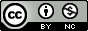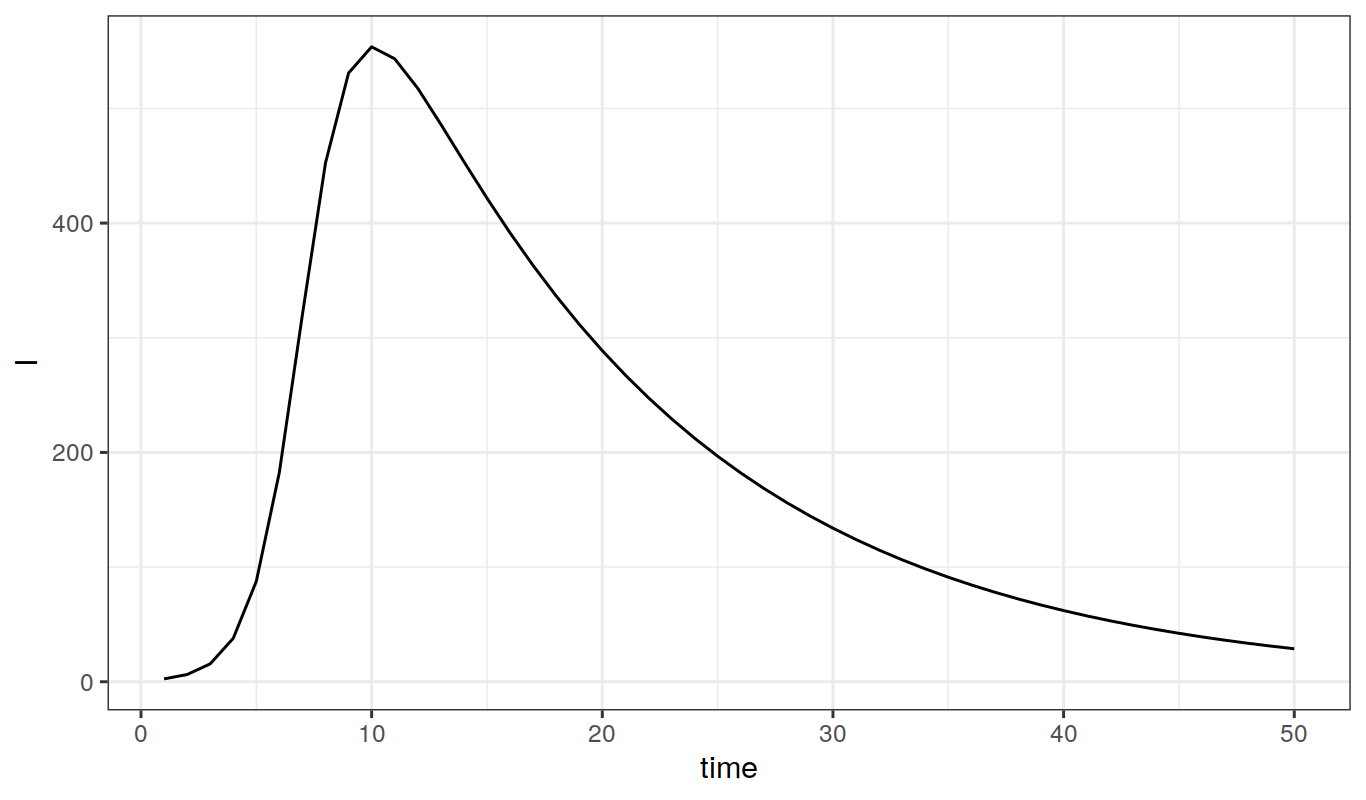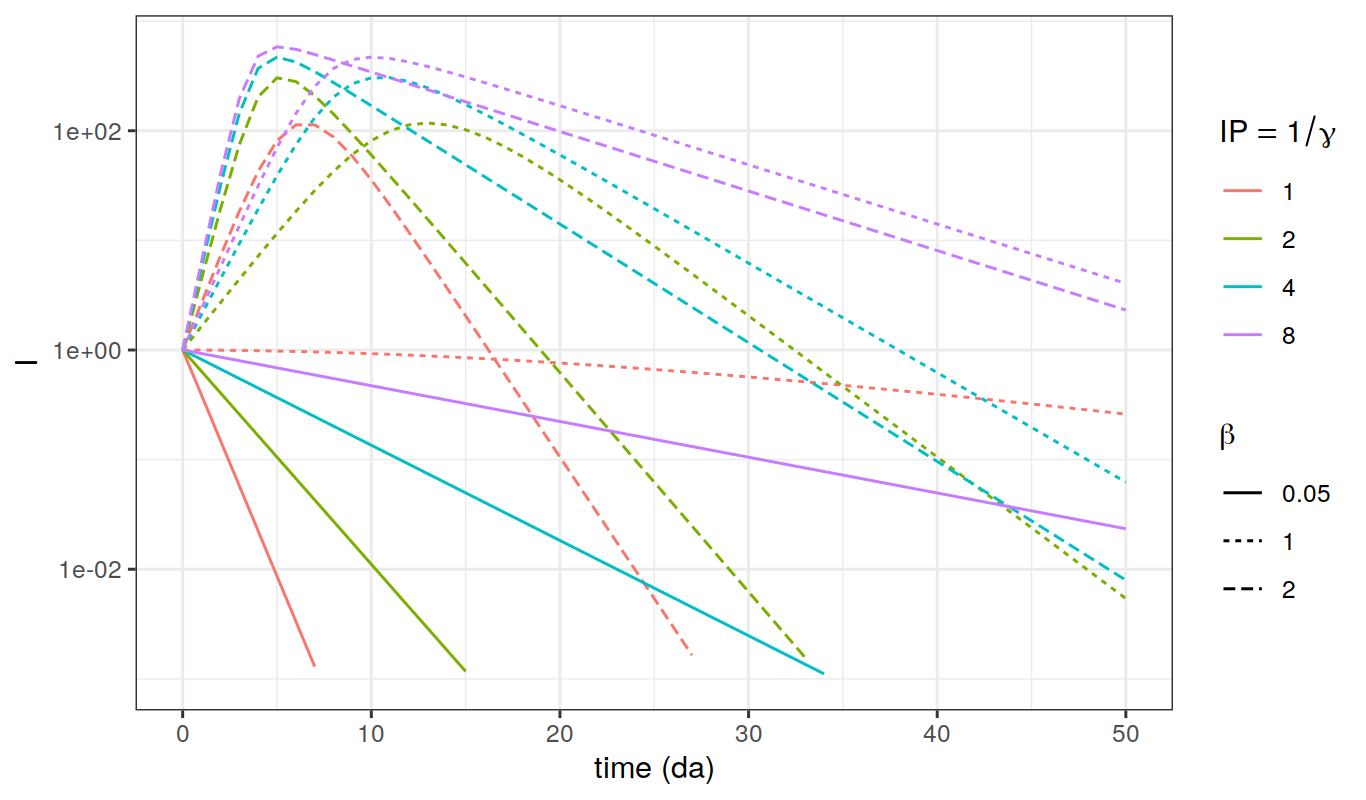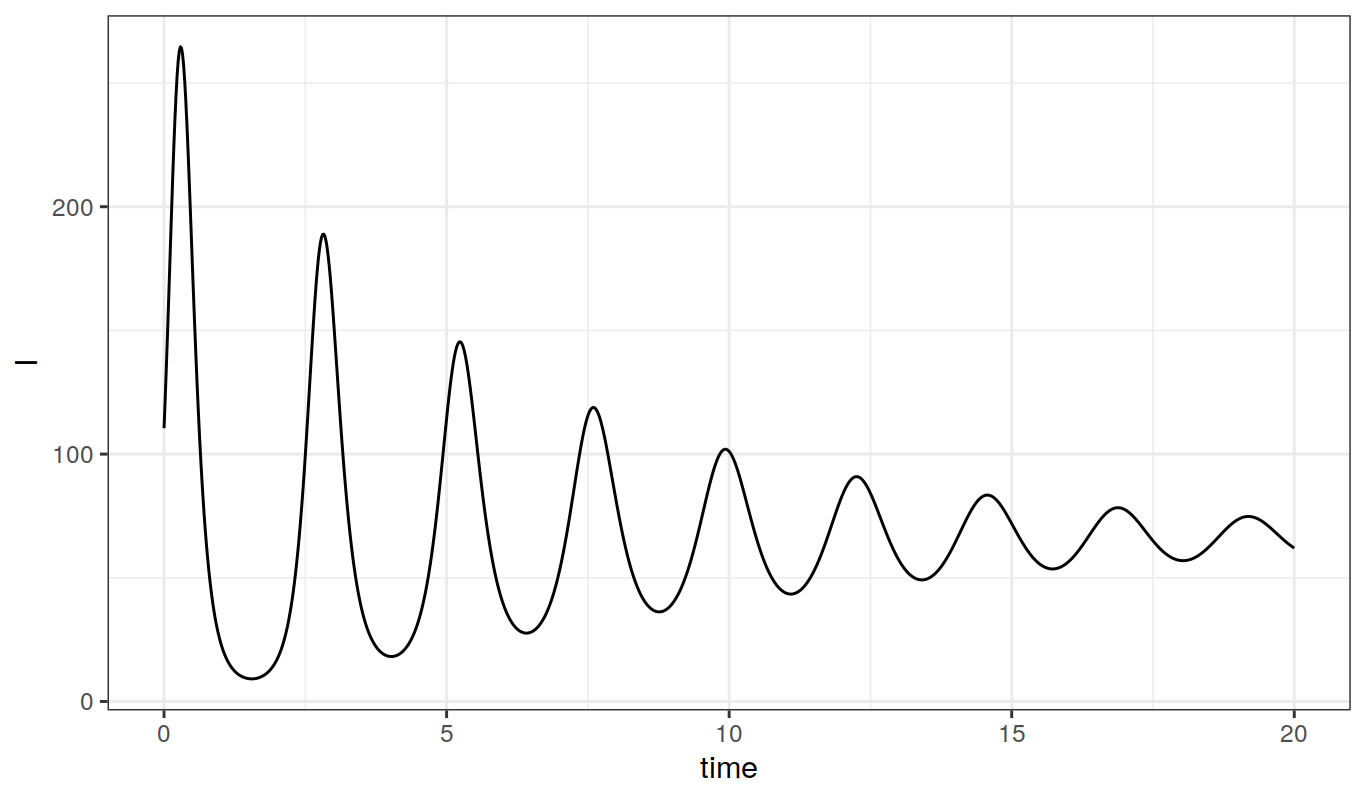Important Note: These materials have been updated for use with version 2.8. As of version 2, pomp syntax has changed substantially. These changes are documented on the pomp website.

Here we begin our study of computational techniques for studying epidemiological models. In this lesson we introduce the numerical solution (or integration) of nonlinear differential equations using the sophisticated solvers incorporated into pomp. Numerical integration is one of the most important tools we have for the analysis of epidemiological models.

### The SIR model

The classical SIR compartmental model divides a population of hosts into three classes: susceptible, infected, recovered. The model describes how the portion of the population in each of these classes changes with time. Births are modeled as flows from “elsewhere” into the susceptible class; deaths are modeled as flows from the S, I, or R compartment into “elsewhere”. If $$S$$, $$I$$, and $$R$$ refer to the numbers of individuals in each compartment, then these state variables change according to the following system of differential equations: \begin{aligned} \frac{dS}{dt} &= B-\lambda\,S-\mu\,S\\ \frac{dI}{dt} &= \lambda\,S-\gamma\,I-\mu\,I\\ \frac{dR}{dt} &= \gamma\,I-\mu\,R.\\ \end{aligned} Here, $$B$$ is the crude birth rate (births per unit time), $$\mu$$ is the death rate and $$\gamma$$ is the recovery rate. We’ll assume that the force of infection, $$\lambda$$, has the form $\lambda = \beta\,\frac{I}{N},$ so that the risk of infection a susceptible faces is proportional to the prevalence (the fraction of the population that is infected). This is known as the assumption of frequency-dependent transmission.

### Numerical integration of ordinary differential equations

Like almost all ecological and epidemiological models, one can’t solve these equations analytically. However, we can compute the trajectories of a continuous-time model such as this one by integrating the equations numerically. Doing this accurately involves a lot of calculation, and there are smart ways and not-so-smart ways of going about it. This very common problem has been very thoroughly studied by numerical analysts for generations so that, when the equations are smooth, well-behaved functions, excellent numerical integration algorithms are readily available to compute approximate solutions to high precision. In particular, R has several sophisticated ODE solvers which (for many problems) will give highly accurate solutions. These are harnessed by pomp. These algorithms are flexible, automatically perform checks, and give informative errors and warnings.

### SIR for a closed epidemic

Let’s study the SIR model for a closed population, i.e., one in which we can neglect births and deaths. Recall that the differential equations for the closed epidemic are \begin{aligned} \frac{dS}{dt} &= -\frac{\beta\,S\,I}{N}\\ \frac{dI}{dt} &= \frac{\beta\,S\,I}{N}-\gamma\,I\\ \frac{dR}{dt} &= \gamma\,I \end{aligned} To incorporate these deterministic equations into a pomp object, we supply them to the pomp function via the skeleton argument as a Csnippet. We must also provide a Csnippet to initialize the state variables $$S$$, $$I$$, and $$R$$. For example:

library(pomp)

closed.sir.ode <- Csnippet("
DS = -Beta*S*I/N;
DI = Beta*S*I/N-gamma*I;
DR = gamma*I;
")

init1 <- Csnippet("
S = N-1;
I = 1;
R = 0;
")

pomp(data=data.frame(time=1:50,data=NA),
times="time",t0=0,
skeleton=vectorfield(closed.sir.ode),
rinit=init1,
statenames=c("S","I","R"),
paramnames=c("Beta","gamma","N")) -> closed.sir

Now we can call trajectory to compute trajectories of the model. To do this, we’ll need some values of the parameters. If we’re thinking of a disease something like measles, and measuring time in days, we might use something like:

params1 <- c(Beta=1,gamma=1/13,N=763)

What is the infectious period of this disease?

Next, we compute a model trajectory with the trajectory command and store the result in a data-frame:

x <- trajectory(closed.sir,params=params1,format="data.frame")

and plot the results using the commands:

library(ggplot2)
ggplot(data=x,mapping=aes(x=time,y=I))+geom_line()##### Exercise: conversion of units

Suppose that you’d rather measure time in years. Modify the parameters accordingly and verify your modifications.

Let’s study how the epidemic curve depends on the transmission rate, $$\beta$$, and the infectious period. In particular, we’ll investigate how the epidemic curve changes as we vary $$\beta$$ from 0.05 to 2 and the infectious period from 1 to 8 days.The ability to numerically integrate ODE is essential, but its power is limited. The next exercise demonstrates the importance of being able to analyze the equations as well.

##### Exercise: exploring the model’s dynamical repertoire

For each of the above parameter combinations, notice that either an epidemic occurs or the infection fades out. Can you predict this behavior from a knowledge of the parameters without numerically integrating the equations?

### The basic reproduction number

A dimensionless quantity of central importance in epidemiology is the so-called basic reproduction number, $$R_0$$, which is the expected number of new infections engendered by a single infected individual introduced into a fully susceptible population. In this case, $$R_0=\frac{\beta}{\gamma}$$, i.e., the product of the transmission rate and the infectious period. Compute $$R_0$$ for each of the parameter combinations you examined in the exercise above and relate it to the presence or absence of an epidemic.

### The epidemic final size

For a simple, closed SIR outbreak, we can derive an expression that determines the final size of the outbreak, i.e., the total number of hosts ultimately infected. To do this, note that if $\begin{equation*}\begin{gathered} \frac{dS}{dt}=-\frac{\beta S I}{N} \qquad \text{and} \qquad \frac{dI}{dt}=\frac{\beta S I}{N}-\gamma\,I, \end{gathered}\end{equation*}$ then $\frac{dI}{dS}=-1+\frac{N}{R_0\,S},$ which we integrate to yield $S(0)-S(\infty)+\frac{N}{R_0}\,\log{\frac{S(\infty)}{S(0)}}=I(\infty)-I(0)=0.$ If $$S(0)=N$$, then $$N-S(\infty)$$ is the final size of the outbreak and the fraction ultimately infected is $$f=\frac{R(\infty)}{N}=1-\frac{S(\infty)}{N}$$. In terms of the latter, we have $R_0=-\frac{\log{(1-f)}}{f}.$

The following shows the relationship between final size and $$R_0$$:##### Exercise: final size

Use trajectory to study the dependence of $$f$$ on $$R_0$$. Compare your results with the predictions of the final size equation $R_0=-\frac{\log{(1-f)}}{f},$ the solution of which is plotted above.

### SIR dynamics in an open population

Over a sufficiently short time scale, the assumption that the population is closed is reasonable. To capture the dynamics over the longer term, we’ll need to account for births and deaths, i.e., allow the population to be an open one. As we’ve seen, if we further assume that the birth rate equals the death rate, then the SIR equations become \begin{aligned} \frac{dS}{dt} &= \mu\,N-\frac{\beta\,S\,I}{N}-\mu\,S\\ \frac{dI}{dt} &= \frac{\beta\,S\,I}{N}-\gamma\,I-\mu\,I\\ \frac{dR}{dt} &= \gamma\,I-\mu\,R\\ \end{aligned}

We must modify the ODE function accordingly:

open.sir.ode <- Csnippet("
DS = -Beta*S*I/N+mu*(N-S);
DI = Beta*S*I/N-gamma*I-mu*I;
DR = gamma*I-mu*R;
")

init2 <- Csnippet("
S = S_0;
I = I_0;
R = N-S_0-I_0;
")

pomp(data=data.frame(time=seq(0,20,by=1/52),cases=NA),
times="time",t0=-1/52,
skeleton=vectorfield(open.sir.ode),
rinit=init2,
statenames=c("S","I","R"),
paramnames=c("Beta","gamma","mu","S_0","I_0","N")
) -> open.sir

We’ll need to specify a birth/death rate in addition to the two parameters we specified before:

params3 <- c(mu=1/50,Beta=400,gamma=365/13,
N=100000,S_0=100000/12,I_0=100)

We integrate the equations as before:

x <- trajectory(open.sir,params=params3,format="d")

We can plot each of the state variables against time, and $$I$$ against $$S$$:

library(ggplot2)
ggplot(data=x,mapping=aes(x=time,y=I))+geom_line()
ggplot(data=x,mapping=aes(x=S,y=I))+geom_path()